# Lecture_10_Parallel_Algorithms_Part_II.ppt

15 Feb 2023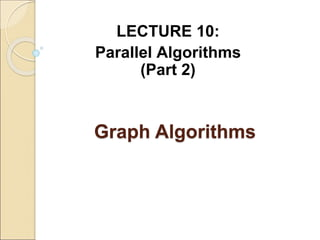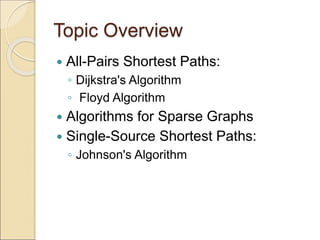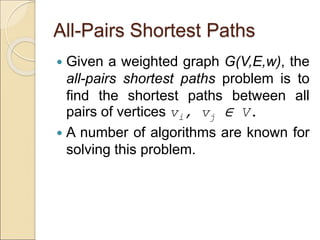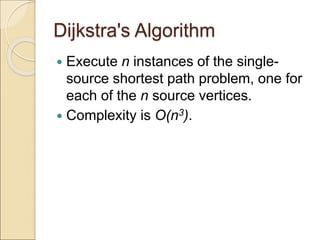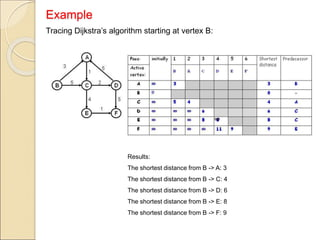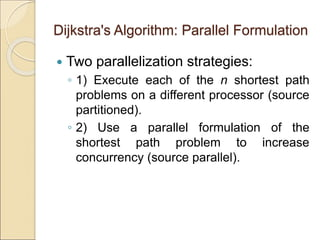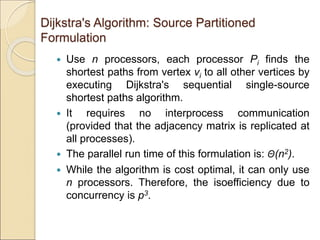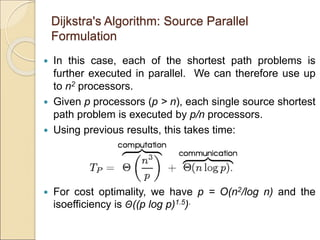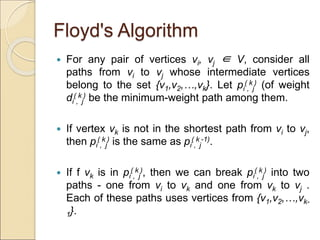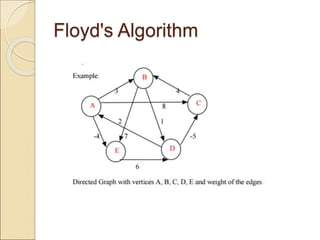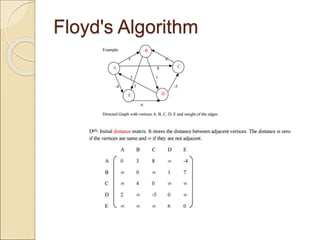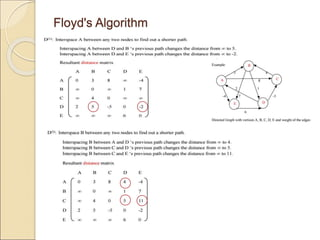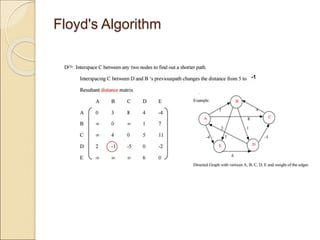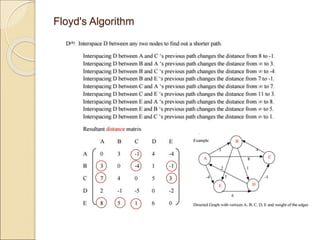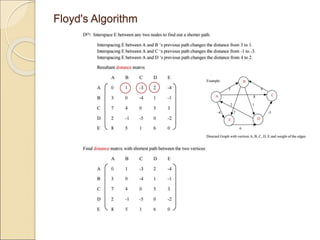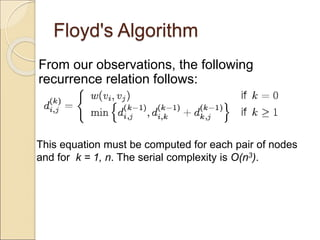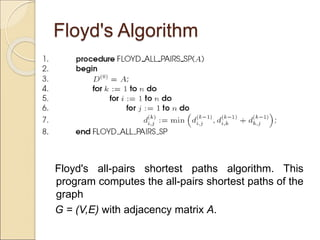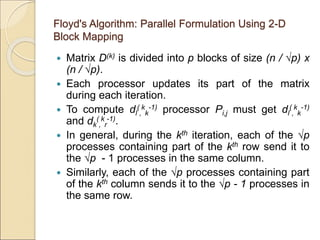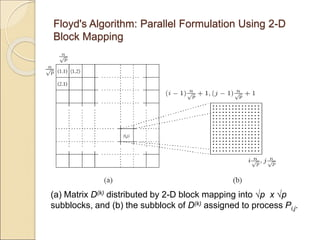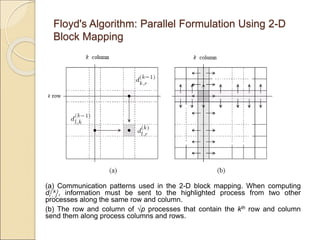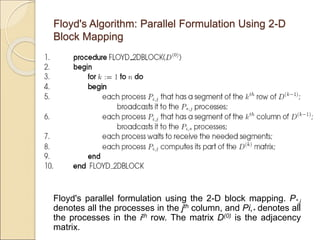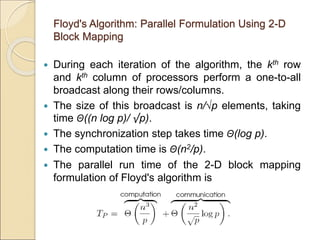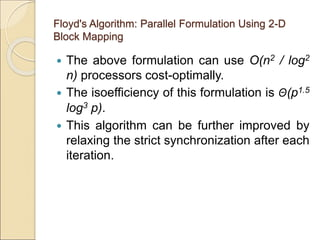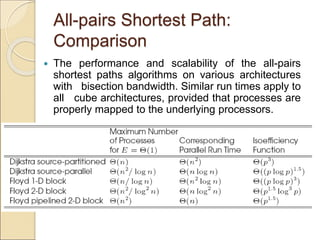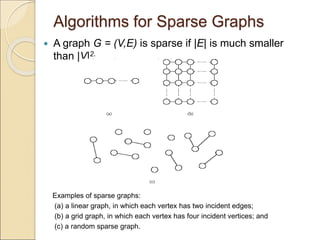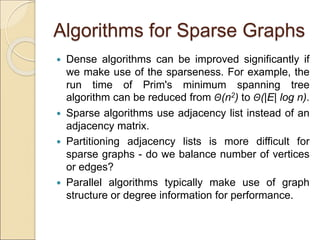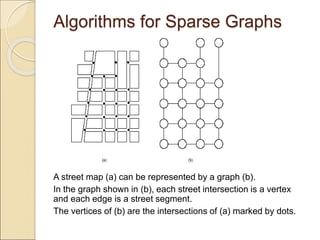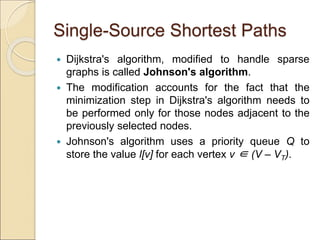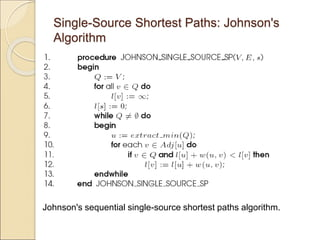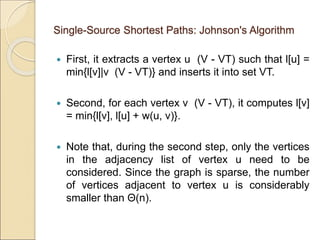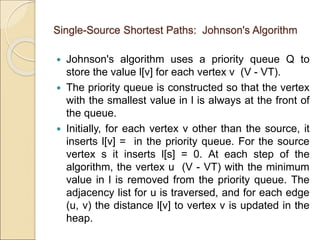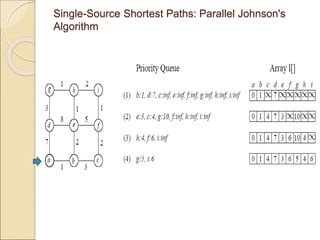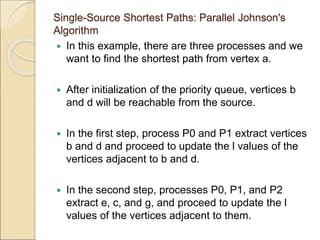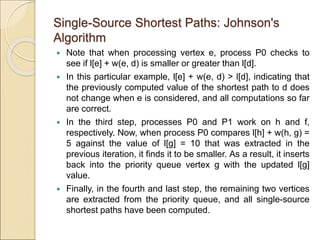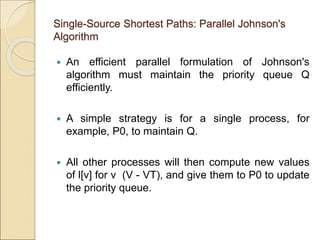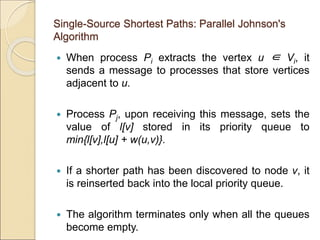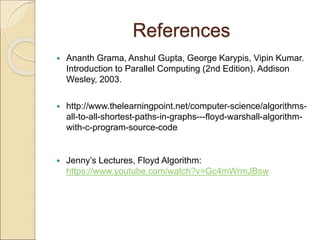1 sur 38

### Lecture_10_Parallel_Algorithms_Part_II.ppt

• 2. Topic Overview  All-Pairs Shortest Paths: ◦ Dijkstra's Algorithm ◦ Floyd Algorithm  Algorithms for Sparse Graphs  Single-Source Shortest Paths: ◦ Johnson's Algorithm
• 3. All-Pairs Shortest Paths  Given a weighted graph G(V,E,w), the all-pairs shortest paths problem is to find the shortest paths between all pairs of vertices vi, vj ∈ V.  A number of algorithms are known for solving this problem.
• 4. Dijkstra's Algorithm  Execute n instances of the single- source shortest path problem, one for each of the n source vertices.  Complexity is O(n3).
• 5. Example Tracing Dijkstra’s algorithm starting at vertex B: Results: The shortest distance from B -> A: 3 The shortest distance from B -> C: 4 The shortest distance from B -> D: 6 The shortest distance from B -> E: 8 The shortest distance from B -> F: 9 ∞
• 6. Dijkstra's Algorithm: Parallel Formulation  Two parallelization strategies: ◦ 1) Execute each of the n shortest path problems on a different processor (source partitioned). ◦ 2) Use a parallel formulation of the shortest path problem to increase concurrency (source parallel).
• 7. Dijkstra's Algorithm: Source Partitioned Formulation  Use n processors, each processor Pi finds the shortest paths from vertex vi to all other vertices by executing Dijkstra's sequential single-source shortest paths algorithm.  It requires no interprocess communication (provided that the adjacency matrix is replicated at all processes).  The parallel run time of this formulation is: Θ(n2).  While the algorithm is cost optimal, it can only use n processors. Therefore, the isoefficiency due to concurrency is p3.
• 8. Dijkstra's Algorithm: Source Parallel Formulation  In this case, each of the shortest path problems is further executed in parallel. We can therefore use up to n2 processors.  Given p processors (p > n), each single source shortest path problem is executed by p/n processors.  Using previous results, this takes time:  For cost optimality, we have p = O(n2/log n) and the isoefficiency is Θ((p log p)1.5).
• 9. Floyd's Algorithm  For any pair of vertices vi, vj ∈ V, consider all paths from vi to vj whose intermediate vertices belong to the set {v1,v2,…,vk}. Let pi ( , k j ) (of weight di ( , k j ) be the minimum-weight path among them.  If vertex vk is not in the shortest path from vi to vj, then pi ( , k j ) is the same as pi ( , k j -1).  If f vk is in pi ( , k j ), then we can break pi ( , k j ) into two paths - one from vi to vk and one from vk to vj . Each of these paths uses vertices from {v1,v2,…,vk- 1}.
• 16. Floyd's Algorithm From our observations, the following recurrence relation follows: This equation must be computed for each pair of nodes and for k = 1, n. The serial complexity is O(n3).
• 17. Floyd's Algorithm Floyd's all-pairs shortest paths algorithm. This program computes the all-pairs shortest paths of the graph G = (V,E) with adjacency matrix A.
• 18. Floyd's Algorithm: Parallel Formulation Using 2-D Block Mapping  Matrix D(k) is divided into p blocks of size (n / √p) x (n / √p).  Each processor updates its part of the matrix during each iteration.  To compute dl ( , k k -1) processor Pi,j must get dl ( , k k -1) and dk ( , k r -1).  In general, during the kth iteration, each of the √p processes containing part of the kth row send it to the √p - 1 processes in the same column.  Similarly, each of the √p processes containing part of the kth column sends it to the √p - 1 processes in the same row.
• 19. Floyd's Algorithm: Parallel Formulation Using 2-D Block Mapping (a) Matrix D(k) distributed by 2-D block mapping into √p x √p subblocks, and (b) the subblock of D(k) assigned to process Pi,j.
• 20. Floyd's Algorithm: Parallel Formulation Using 2-D Block Mapping (a) Communication patterns used in the 2-D block mapping. When computing di ( , k j ), information must be sent to the highlighted process from two other processes along the same row and column. (b) The row and column of √p processes that contain the kth row and column send them along process columns and rows.
• 21. Floyd's Algorithm: Parallel Formulation Using 2-D Block Mapping Floyd's parallel formulation using the 2-D block mapping. P*,j denotes all the processes in the jth column, and Pi,* denotes all the processes in the ith row. The matrix D(0) is the adjacency matrix.
• 22. Floyd's Algorithm: Parallel Formulation Using 2-D Block Mapping  During each iteration of the algorithm, the kth row and kth column of processors perform a one-to-all broadcast along their rows/columns.  The size of this broadcast is n/√p elements, taking time Θ((n log p)/ √p).  The synchronization step takes time Θ(log p).  The computation time is Θ(n2/p).  The parallel run time of the 2-D block mapping formulation of Floyd's algorithm is
• 23. Floyd's Algorithm: Parallel Formulation Using 2-D Block Mapping  The above formulation can use O(n2 / log2 n) processors cost-optimally.  The isoefficiency of this formulation is Θ(p1.5 log3 p).  This algorithm can be further improved by relaxing the strict synchronization after each iteration.
• 24. All-pairs Shortest Path: Comparison  The performance and scalability of the all-pairs shortest paths algorithms on various architectures with bisection bandwidth. Similar run times apply to all cube architectures, provided that processes are properly mapped to the underlying processors.
• 25. Algorithms for Sparse Graphs  A graph G = (V,E) is sparse if |E| is much smaller than |V|2. Examples of sparse graphs: (a) a linear graph, in which each vertex has two incident edges; (b) a grid graph, in which each vertex has four incident vertices; and (c) a random sparse graph.
• 26. Algorithms for Sparse Graphs  Dense algorithms can be improved significantly if we make use of the sparseness. For example, the run time of Prim's minimum spanning tree algorithm can be reduced from Θ(n2) to Θ(|E| log n).  Sparse algorithms use adjacency list instead of an adjacency matrix.  Partitioning adjacency lists is more difficult for sparse graphs - do we balance number of vertices or edges?  Parallel algorithms typically make use of graph structure or degree information for performance.
• 27. Algorithms for Sparse Graphs A street map (a) can be represented by a graph (b). In the graph shown in (b), each street intersection is a vertex and each edge is a street segment. The vertices of (b) are the intersections of (a) marked by dots.
• 28. Single-Source Shortest Paths  Dijkstra's algorithm, modified to handle sparse graphs is called Johnson's algorithm.  The modification accounts for the fact that the minimization step in Dijkstra's algorithm needs to be performed only for those nodes adjacent to the previously selected nodes.  Johnson's algorithm uses a priority queue Q to store the value l[v] for each vertex v ∈ (V – VT).
• 29. Single-Source Shortest Paths: Johnson's Algorithm Johnson's sequential single-source shortest paths algorithm.
• 30. Single-Source Shortest Paths: Johnson's Algorithm  First, it extracts a vertex u (V - VT) such that l[u] = min{l[v]|v (V - VT)} and inserts it into set VT.  Second, for each vertex v (V - VT), it computes l[v] = min{l[v], l[u] + w(u, v)}.  Note that, during the second step, only the vertices in the adjacency list of vertex u need to be considered. Since the graph is sparse, the number of vertices adjacent to vertex u is considerably smaller than Θ(n).
• 31. Single-Source Shortest Paths: Johnson's Algorithm  Johnson's algorithm uses a priority queue Q to store the value l[v] for each vertex v (V - VT).  The priority queue is constructed so that the vertex with the smallest value in l is always at the front of the queue.  Initially, for each vertex v other than the source, it inserts l[v] = in the priority queue. For the source vertex s it inserts l[s] = 0. At each step of the algorithm, the vertex u (V - VT) with the minimum value in l is removed from the priority queue. The adjacency list for u is traversed, and for each edge (u, v) the distance l[v] to vertex v is updated in the heap.
• 32. Single-Source Shortest Paths: Parallel Johnson's Algorithm
• 33. Single-Source Shortest Paths: Parallel Johnson's Algorithm  In this example, there are three processes and we want to find the shortest path from vertex a.  After initialization of the priority queue, vertices b and d will be reachable from the source.  In the first step, process P0 and P1 extract vertices b and d and proceed to update the l values of the vertices adjacent to b and d.  In the second step, processes P0, P1, and P2 extract e, c, and g, and proceed to update the l values of the vertices adjacent to them.
• 34. Single-Source Shortest Paths: Johnson's Algorithm  Note that when processing vertex e, process P0 checks to see if l[e] + w(e, d) is smaller or greater than l[d].  In this particular example, l[e] + w(e, d) > l[d], indicating that the previously computed value of the shortest path to d does not change when e is considered, and all computations so far are correct.  In the third step, processes P0 and P1 work on h and f, respectively. Now, when process P0 compares l[h] + w(h, g) = 5 against the value of l[g] = 10 that was extracted in the previous iteration, it finds it to be smaller. As a result, it inserts back into the priority queue vertex g with the updated l[g] value.  Finally, in the fourth and last step, the remaining two vertices are extracted from the priority queue, and all single-source shortest paths have been computed.
• 35. Single-Source Shortest Paths: Parallel Johnson's Algorithm  An efficient parallel formulation of Johnson's algorithm must maintain the priority queue Q efficiently.  A simple strategy is for a single process, for example, P0, to maintain Q.  All other processes will then compute new values of l[v] for v (V - VT), and give them to P0 to update the priority queue.
• 36. Single-Source Shortest Paths: Parallel Johnson's Algorithm  When process Pi extracts the vertex u ∈ Vi, it sends a message to processes that store vertices adjacent to u.  Process Pj, upon receiving this message, sets the value of l[v] stored in its priority queue to min{l[v],l[u] + w(u,v)}.  If a shorter path has been discovered to node v, it is reinserted back into the local priority queue.  The algorithm terminates only when all the queues become empty.
• 37. References  Ananth Grama, Anshul Gupta, George Karypis, Vipin Kumar. Introduction to Parallel Computing (2nd Edition). Addison Wesley, 2003.  http://www.thelearningpoint.net/computer-science/algorithms- all-to-all-shortest-paths-in-graphs---floyd-warshall-algorithm- with-c-program-source-code  Jenny’s Lectures, Floyd Algorithm: https://www.youtube.com/watch?v=Gc4mWrmJBsw